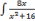# Mathematics Sample Question (Secondary Level):Teacher Service Commission

December 30, 2021

Government of Nepal Teachers Service Commission

Open Competitive Examination 2078

Subject: Mathematics Sample Question

Level: Secondary Full Marks:100 Pass Marks: 40 Time: 3hrs

Attempt all questions

### Section A

1. It is learnt that most of the students feel difficulties to understand and apply the concepts of statistics and probabilities in real life. Considering these situations, answer the  following questions:

a. How do you explain students about the use of measure of dispersion like range,  coefficient of range, mean deviation, standard deviation, coefficient of variation in real life?

b. How do you clarify and use the relationship and difference between conditional  probability and Baye’s theorem while teaching in your classroom?

2. According to Bruner's theory of cognitive development, any subject can be taught effectively in some intellectually honest form to any child at any stage of development. In this scenario, you are given to teach reflection transformation in grade 9. Discuss through  appropriate activities how three modes of representations – concrete (enactive), semi concrete (iconic) and abstract (Symbolic) can be used. Also make one test items of comprehension, application and higher ability each according to Bloom's taxonomy from  this lesson. [7+3]

3. While teaching linear programming, students can solve how to maximize the given objective function but they don't understand its real-life implementation. In this context, a  problem is given as follows:

A company produces two types of pots X and Y by using copper and Steel. Type X pot requires 300 g of copper and 100 g of steel while Y requires 100 gram of copper and 200  grams of steel. The X pot brings profit of Rs. 400 and the Y pot brings profit of Rs. 500.  Find the number of units of each type of pots that the company should produce to achieve maximum profit.

How do you make your students understand and let them develop its objective function,  constraints, and maximum profit that the company can make with the given situation?  Prepare your form of teaching-learning activities. While writing your activities, you are suggested to analyze the possible error that students can make and implement your strategy to overcome those errors during teaching. [6+4]

### Section B

4. There are different types of geometries, such as Euclidean, hyperbolic and elliptic. The topological study of solid figures give rise to very useful relations between vertex, edge  and face. Given these situations, respond to the following questions:

a. Explain how the sum of angles of a triangle are equal to, less than, and more than two right angles based on the assumptions of Euclidian and non-Euclidean geometry. 

b. Show through an example that for any convex polyhedron, V – E + F = 2 where V  is the number of vertices, E is the number of edges and F is the number of faces. 

5. Illustrate derivative and anti derivative geometrically and write one example each where these concepts are used. Find. [6+4]

6. Justify with suitable examples that there has been a big shift in the past and present  education trends especially in terms of objectives, contents, methods, assessment and  research in mathematics teaching and learning. 

द्रष्टव्य: पाठ्यक्रमको खण्ड क बाट पूर्णाङ्क ५० का ५ प्रश्न र खण्ड ख बाट पूर्णाङ्क ५० का ५ प्रश्न गरी १०० पूर्णाङ्कको परीक्षाका लागि यस्तै प्रकृतिका प्रश्नहरू दिइने छ ।

• 01-6637873, 01-6638151
• tscapplication1@gmail.com